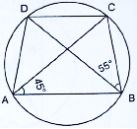# Chapter 16 Circles RD Sharma Solutions Exercise 16.5 Class 9 Maths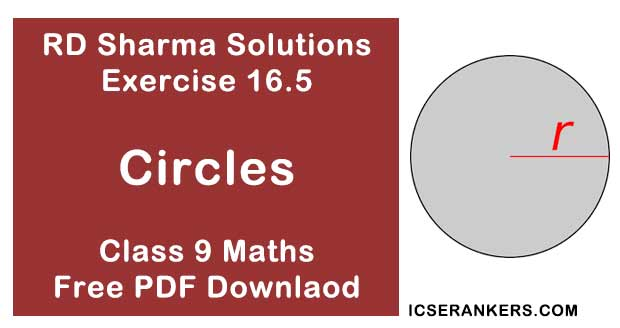Chapter Name RD Sharma Chapter 16 Circles Exercise 16.5 Book Name RD Sharma Mathematics for Class 10 Other Exercises Exercise 16.1Exercise 16.2Exercise 16.3Exercise 16.4 Related Study NCERT Solutions for Class 10 Maths

### Exercise 16.5 Solutions

1. In the below fig. ΔABC is an equilateral triangle. Find in ∠BEC.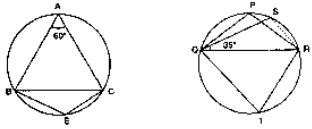Solution

Since, ΔABC is an equilateral triangles
Then, ∠BAC = 60°
∴ ∠BAC + ∠BEC = 180° [Opposite angles of a quadrilaterals]
⇒ 60° + ∠BEC = 180°
⇒ ∠BEC = 180° - 60°
⇒ ∠BEC = 180°

2. In the below fig. ΔPQR is an isosceles triangle with PQ = PR and m ∠PQR = 35° . Find m ∠QSR and m ∠QTR.Solution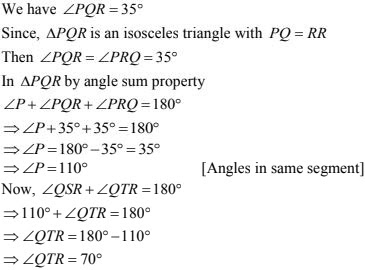3. In the below fig., O is the centre of the circle. If ∠BOD = 160°, find the values of x and y.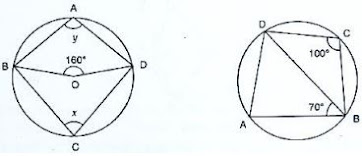Solution

Given that O is the centre of the circle
We have, ∠BOD = 160°
By degree measure theorem
∠BOD = 2∠BCD
⇒ 160° = 2 × x
⇒ x = 160/2 = 80°
⇒ y + x = 180°
⇒ y + 80° = 180°
⇒ y = 180° - 80° = 100°

4. In the below fig. ABCD is a cyclic quadrilateral. It ∠BCD = 100° and ∠ABD = 70°, find ∠ADB.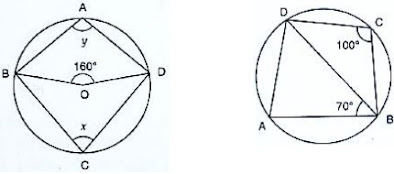Solution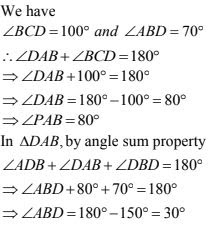5. If ABCD is a cyclic quadrilateral in which AD || BC (Fig. below). Prove that ∠B = ∠C.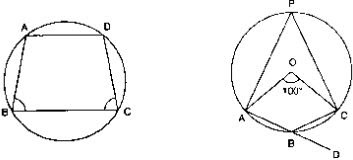Solution

Then ∠A + ∠C = 180° ... (1)  [Opposite angles of cyclic quadrilaterals]
And, ∠A + ∠B = 180° ...(2) [Co interior angles]
Compare (1) and (2) equations ∠B = ∠C

6. In the below fig. O is the centre of the circle. Find ∠CBD.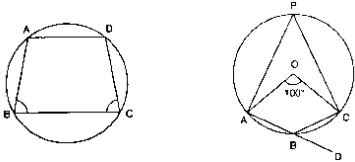Solution
Given that ∠BOC = 100°
By degree measure theorem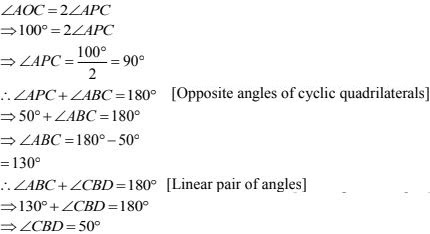7. In the below fig. AB and CD are diameters of a circle with centre O. if ∠OBD = 50°, find ∠AOC.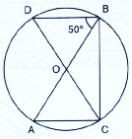Solution
Given that,
∠OBD = 50°
Since, AB and CD are the diameter of circle then O is the center of the circle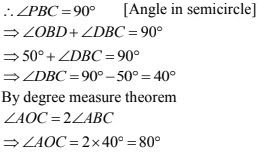8. On a semi - circle with AB as diameter, a point C is a taken, so that m (∠CAB) = 30°. Find m (∠ACB) and m(∠ABC).
Solution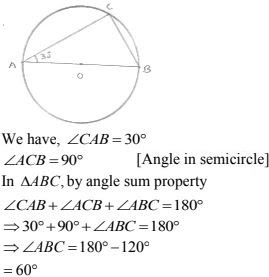9. In a cyclic quadrilateral ABCD if AB || CD and ∠B = 70°, find the remaining angles.
Solution
Given that ∠B = 70° = 70°
Since ABCD is a cyclic quadrilaterals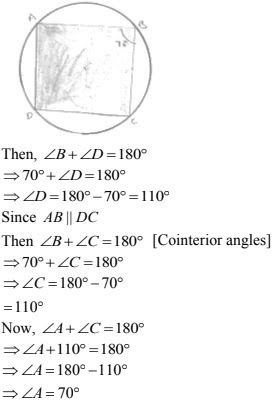10. In a quadrilateral ABCD, if m ∠A = 3 (m ∠C). Find m ∠A.
Solution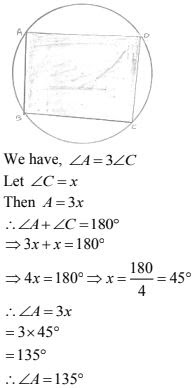11. In the below fig. O is the centre of the circle and ∠DAB = 50°. Calculate the values of x and y.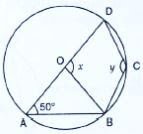Solution
We have ∠DAB = 50°
By degree measure theorem
⇒ x = 2 × 50 = 100°
Since, ABCD is a cyclic quadrilateral
Then ∠A + ∠C = 180 °
⇒ 50 + y = 180°
⇒ y = 180° - 50° = 130°

12. In the below fig. in ∠BAC = 60° and ∠BCA = 20° , find ∠ADC.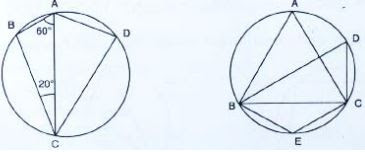Solution
By using angle sum property in ΔABC
∠B = 180° - (60° + 20°) = 100°
In cyclic quadrilaterals ABCD, we have :
∠B + ∠D = 180°
∠D = 180° - 100° = 80°

13. In the below fig. if ABC is an equilateral triangle. Find ∠BDC and ∠BEC.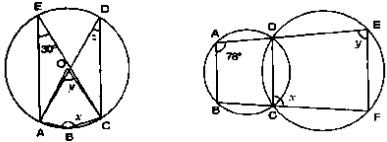Solution
Since ΔABC is an equilateral triangle
Then, ∠BAC = 60°
∴ ∠BDC = ∠BAC = 60°  [Angles in same segment]
Then ∠BAC + ∠BEC = 180°
⇒ 60° + ∠BEC = 180°
⇒ ∠BEC = 180° - 60° = 120°

14. In the below fig. O is the centre of the circle, if ∠CEA = 30°, find the values of x, y and z.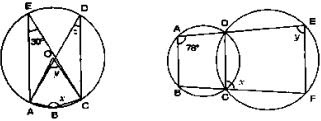Solution
We have ∠AEC = 30°
Then, ∠ABC + ∠AEC = 180°
x + 30° = 180°
⇒ x = 180 - 30 = 150°
By degree measure theorem
∠AOC = 2∠AEC
⇒ y = 2× 30° = 60°
⇒ ∴ ∠ADC = ∠AEC  [Angles in same segment]

15. In the below fig. ∠BAD = 78° , ∠DCF = x° and ∠DEF = y° . Find the values of x, and y.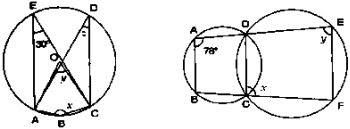Solution
We have, ∠BAD = 78°, ∠DCF = x° and ∠DEF = y°
Since, ABCD is a cyclic quadrilateral
Then, ∠BAD + ∠BCD = 180°
⇒ 78° + ∠BCD = 180°
⇒ ∠BCD = 180° - 78° = 102°
Now, ∠BCD + ∠DCF = 180° [Linear pair of angles]
⇒ 102° = x - 180°
⇒ x = 180° - 102° = 78°
Since, DCEF is a cyclic quadrilateral
Then, x + y = 180°
⇒ 78° + y = 180°
⇒ y = 180° - 78° = 102°
∴ y = 102°

16. In a cyclic quadrilateral ABCD, if ∠A - ∠C = 60° , prove that the smaller of two is 60° .
Solution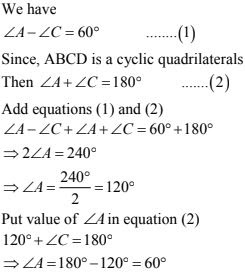17. In the below fig. ABCD is a cyclic quadrilateral. Find the value of x.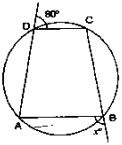Solution18. ABCD is a quadrilateral in which :
(i) BC || AD, ∠ADC = 110° and ∠BAC = 50° . Find ∠DAC.
(ii) ∠DBC = 80° and ∠BAC = 40°, find ∠BCD.
(iii) ∠BCD = 100° and ∠ABD = 70°, find ∠ADB.
Solution
(i)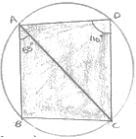Since, ABCD is a cyclic quadrilateral
Then, ∠ABC + 110° = 180°
⇒ ∠ABC + 110° = 180°
⇒ ∠ABC = 180° - 110° = 70°
Then, ∠DAB + ∠ABC = 180°  [Co - interior angle]
⇒ ∠DAC + 50° + 70° = 180°
⇒ ∠DAC = 180° - 120° = 60°

(ii) ∠BAC = ∠BDC = 40°  [Angle in same segment]
In ΔBDC, by angle sum property
∠DBC + ∠BCD + ∠BDC = 180°
⇒ 80° + ∠BCD + 40° = 180°
⇒ ∠BCD = 180° - 40° - 80°
⇒ ∠BCD = 60°

(iii) Given that ABCD is a cyclic quadrilaterals
Ten ∠BAD + ∠BCD = 180°
⇒ ∠BAD  = 180° - 100°
In ΔABD, by angle sum property
⇒ 70° + ∠ADB + 80° = 180°
⇒ ∠ADB = 180° - 150°

19. Prove that the perpendicular bisectors of the sides of a cyclic quadrilateral are concurrent.
Solution
Let ABCD be a cyclic quadrilateral, and let O be the center of the corresponding circle.
Then, each side of the equilateral ABCD is a chord of the circle and the perpendicular
bisector of a chord always passes through the center of the circle.
So, right bisectors of the sides of quadrilaterals ABCD, will pass through the circle O of the
corresponding circle

20. Prove that the centre of the circle circumscribing the cyclic rectangle ABCD is the point of intersection of its diagonals.
SolutionLet O be the circle circumscribing the cycle rectangle ABCD. Since ∠ABC = 90° and AC is a chord of the circle, so AC is a diameter of a circle. Similarly BD is a diameter
Hence, point of intersection of AC and BD is the center of the circle

21. Prove that the circles described on the four sides of a rhombus as diameters, pass through the point of intersection of its diagonals.
Solution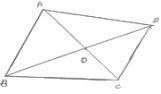Let ABCD be a rhombus such that its diagonals AC and BD interest at O
Since, the diagonals of a rhombus intersect at right angle
∴ ∠ACB = ∠BOC = ∠COD = ∠DOA = 90°
Now, ∠AOB = 90°
⇒ circle described on BC, AD and CD as diameter pass through O.

22. If the two sides of a pair of opposite sides of a cyclic quadrilateral are equal, prove that its diagonals are equal.
Solution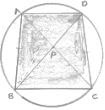Given ABCD is a cyclic quadrilateral in which AB = DC
To prove : AC = BD
Proof : In ΔPAB and ΔPDC
Given that AB = DC
∠BAD  = ∠CDP  [Angles in the same segment]
∠PBA = ∠PCD  [Angles in same segment]
Then ΔPAB = ΔPDC  ...(1) [c.p.c.t]
PC = PB  ...(2) [c.p.c.t]
PA + PC = PD + PB
⇒ AC = BD

23. ABCD is a cyclic quadrilateral in which BA and CD when produced meet in E and EA = ED. Prove that
(ii) EB = EC
Solution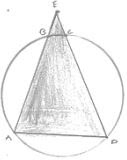Given ABCD is a cyclic quadrilateral in which EA = ED
To prove:
(ii) EB = EC
Proof:
(i) Since EA = ED
Then ∠EAD = ∠EDA  [Opposite angles to equal sides]
Since, ABCD is a cyclic quadrilaterals
Then, ∠ABC + ∠ADC = 180°
But ∠ABC + ∠EBC = 180°  [Linear pair of angles]
Compare equation (1) and (2)
Since, corresponding angle are equal

(ii) From equation (2)
Since, corresponding angle are equal
(ii) From equation (2)
Similarly ∠EDA = ∠ECB ...(4)
Compare equation (1), (3) and (4) ∠EBC  = ∠ECD
⇒ EB = EC  (Opposite angles to equal sides)

24. Circles are described on the sides of a triangle as diameters. Prove that the circles on any
two sides intersect each other on the third side (or third side produced).
Solution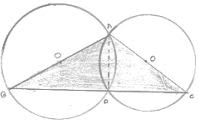Since AB is a diameter
Then ∠ADB = 90°  ...(1) [Angle in semicircle]
Since AC is a diameter
Then ∠ADC = 90°  ...(2) [Angle in semicircle]
⇒ ∠BDC = 180°
Then, BDC is a line
Hence, the circles on any two sides intersect each other on the third side

25. Prove that the angle in a segment shorter than a semicircle is greater than a right angle.
Solution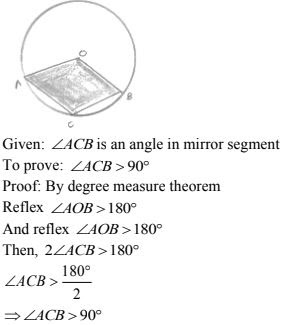27. ABCD is a cyclic trapezium with AD || BC. If ∠B = 70° , determine other three angles of the trapezium.
Solution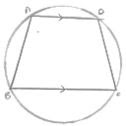Given that
ABCD is a cyclic trapezium with AD || BC and ∠B = 70°
Then ∠B + ∠D = 180°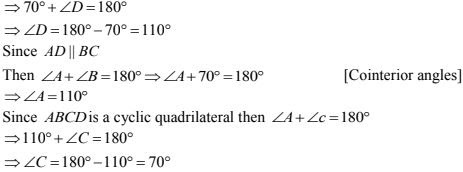28. Prove that the line segment joining the mid - point of the hypotenuse of a right triangle to its opposite vertex is half of the hypotenuse.
Solution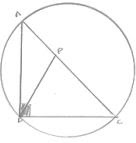Let ΔABC be a right angle triangle at angle B.
Let P be the midpoint of hypotenuse AC.
Draw a circle with center P and AC as a diameter
Since, ∠ABC = 90° , therefore the circle passes through B